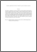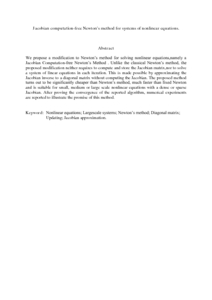# Jacobian computation-free Newton's method for systems of nonlinear equations.

## Citation

Yusuf, Mohammed Waziri and Leong, Wah June and Abu Hassan, Malik and Monsi, Mansor (2010) Jacobian computation-free Newton's method for systems of nonlinear equations. Journal of Numerical Mathematics and Stochastics, 2 (1). pp. 54-63. ISSN 2151-2302

## Abstract

We propose a modification to Newton’s method for solving nonlinear equations,namely a Jacobian Computation-free Newton’s Method . Unlike the classical Newton’s method, the proposed modification neither requires to compute and store the Jacobian matrix,nor to solve a system of linear equations in each iteration. This is made possible by approximating the Jacobian inverse to a diagonal matrix without computing the Jacobian. The proposed method turns out to be significantly cheaper than Newton’s method, much faster than fixed Newton and is suitable for small, medium or large scale nonlinear equations with a dense or sparse Jacobian. After proving the convergence of the reported algorithm, numerical experiments are reported to illustrate the promise of this method.Preview
PDF (Abstract)
Jacobian computation-free Newton's method for systems of nonlinear equations..pdfView Item